# Why “number of parameters” isn’t good enough

A friend of mine recently pointed out a curious fact. Any set of two-dimensional data whatsoever can be perfectly fit by a simple two-parameter sinusoidal model.

y(x) = A sin(Bx)

Sound wrong? Check it out:Zoomed out:N = 10 points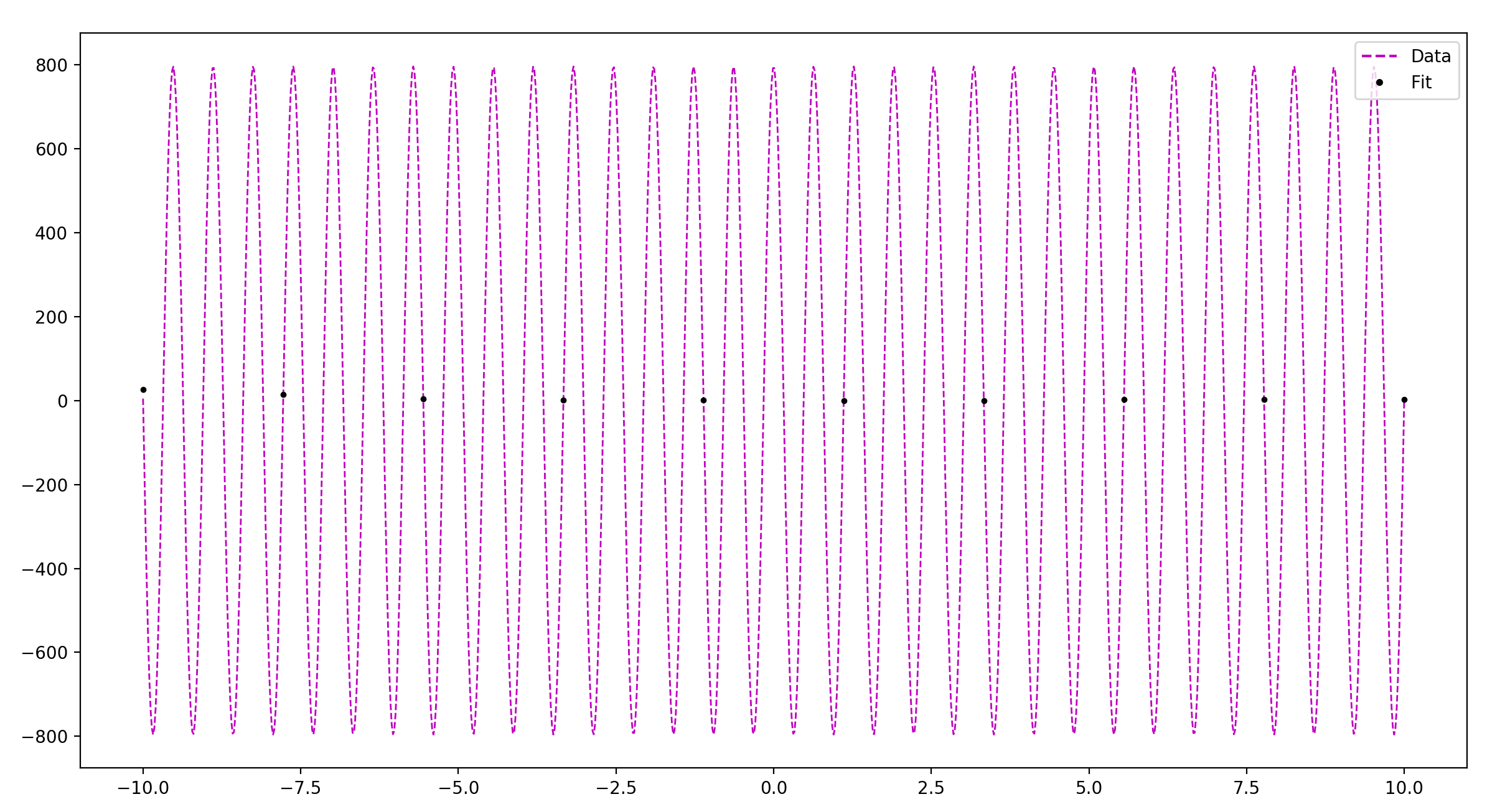As you see, as the number of data points goes up, all you need to do to accommodate this is increase the frequency in your sine function, and adjust the amplitude as necessary. Ultimately, you can fit any data set with a ridiculously quickly oscillating and large-amplitude sine function.

Now, most model selection methods explicitly rely on the parameter count to estimate the potential of a model to overfit. For example, if k is the number of parameters in a model, and L is the log likelihood of the data given the model, we have:

AIC = L – k
BIC = L – k/2・log(N)

This little example represents a fantastic failure of parameter count to successfully do the job AIC and BIC ask of it. Evidently parameter count is too blunt an instrument to do the job we require of it, and we need something with more nuance.

One more example.

For any set of data, if you can perfectly fit a curve to each data point, and if your measurement error σ is an adjustable parameter, then you can take the measurement error to zero to have a fit with infinite accuracy. Now when we evaluate, you find it running off to infinity! Thus our ‘fit to data’ term L goes to infinity, while the model complexity penalty stays a small finite number.

Once again, we see the same lack of nuance dragging us into trouble. The number of parameters might do well at estimating overfitting potential for some types of well-behaved parameters, but it clearly doesn’t do the job universally. What we want is some measure that is sensitive to the potential for some parameters to capture “more” of the space of all possible distributions than others.

And lo and behold, we have such a measure! This is the purpose of information geometry and the volume of a model in the space formed by the Fisher information metric as the penalty for overfitting potential. You can learn more about it in a post I wrote here.

# Bayesian Occam’s Razor

A couple of days ago I posted a question that has been bugging me; namely, does Bayes’ overfit, and if not, why not?

Today I post the solution!

There are two parts: first, explaining where my initial argument against Bayes went wrong, and second, describing the Bayesian Occam’s Razor, the key to understanding how a Bayesian deals with overfitting.

# Part 1: Why I was wrong

Here’s the argument I wrote initially:

1. Overfitting arises from an excessive focus on accommodation. (If your only epistemic priority is accommodating the data you receive, then you will over-accommodate the data, by fitting the noise in the data instead of just the underlying trend.)
2. We can deal with overfitting by optimizing for other epistemic virtues like simplicity, predictive accuracy, or some measure of distance to truth. (For example, minimum description length and maximum entropy optimize for simplicity, and cross validation optimizes for predictive accuracy).
3. Bayesianism is an epistemological procedure that has two steps, setting of priors and updating those priors.
4. Updating of priors is done via Bayes’ rule, which rewards theories according to how well they accommodate their data (creating the potential for overfitting).
5. Bayesian priors can be set in ways that optimize for other epistemic virtues, like simplicity or humility.
6. In the limit of infinite evidence, differences in priors between empirically distinguishable theories are washed away.
7. Thus, in the limit, Bayesianism becomes a primarily accommodating procedure, as the strength of the evidential update swamps your initial differences in priors.

Here’s a more formal version of the argument:

1. The relative probabilities of two model given data is calculated by Bayes’ rule:
P(M | D) / P(M’ | D)  = P(M) / P(M’)・P(D | M) / P(D | M’)
2. If M overfits the data and M’ does not, then as the size of the data set |D| goes to infinity, the likelihood factor P(D | M) / P(D | M’) goes to infinity.
3. Thus the posterior probability P(M | D) should go to 1 for the model that most drastically overfits the data.

This argument is wrong for a couple of reasons. For one, the argument assumes that as the size of the data set grows, the model stays the same. But this is very much not going to be true in general. The task of overfitting gets harder and harder as the number of data points go up. It’s not that there’s no longer noise in the data; it’s that the signal becomes more and more powerful.

A perfect polynomial fit on 100 data points must have, at the worst, 100 parameters. On 1000 data points: 1000 parameters. Etc. In general, as you add more data points, a model that was initially overfitting (e.g. the 100-parameter distribution) will find that it is harder and harder to ignore the signal for the noise, and the next best overfitting model will have more parameters (e.g. the 1000-parameter distribution).

But now we have a very natural solution to the problem we started with! It is true that as the number of data points increases, the evidential support for the model that overfits the data will get larger and larger. It’s also true is that the number of parameters required to overfit the data will grow as well. So if your prior in a model is a decreasing function of the number of parameters in the model, then you can in principle find a perfect balance and avoid overfitting. This perfect balance would be characterized by the following: each time you increase the number of parameters, the prior should decrease by an amount proportional to how much more you get rewarded by overfitting the data with the extra parameters.

How do we find this prior in practice? Beats me… I’d be curious to know, myself.

But what’s most interesting to me is that to solve overfitting as a Bayesian, you don’t even need the priors; the solution comes from the evidential update! It turns out that in fact, the likelihood function for updating credences in a model given data automatically incorporates in model overparameterization. Which brings us to part 2!

# Part 2: Bayesian Occam’s Razor

That last sentence bears repeating. In reality, although priors can play some role by manually penalizing models with high overfitting potential, the true source of the Bayesian Occam’s razor comes from the evidential update. What we’ll find by the end of this post is that models that overfit don’t actually get a stronger evidential update than models that don’t.

You might wonder how this is possible. Isn’t it practically the definition of overfitting that it is an enhancement of the strength of an evidential update through fitting to noise in the data?

Sort of. It is super important to keep in mind the distinction between a model and a distribution. A distribution is a single probability function over your possible observable data. A model is a set of distributions, characterized by a set of parameters. When we say that some models have the potential to overfit a set of data, what we are really saying is that some models contain distributions that overfit the data.

Why is this important? Because assessing the posterior probability of the model is not the same as assessing the posterior probability of the overfitting distribution within the model! Here’s Bayes’ rule, applied to the model and to the overfitting distribution:

(1) P(M | D) = P(M)・P(D | M) / P(D)

(2) P(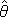| D) = P()・P(D |) / P(D)

It’s clear how to evaluate equation (2). You have some prior probability assigned to, you know how to assess the likelihood function P(D |), and P(D) is an integral that is in principle do-able. In addition, equation (2) has the scary feature we’ve been talking about: the likelihood function P(D |) is really really large if our parameteroverfits the data, potentially large enough to swamp the priors and screw up our Bayesian calculus.

But what we’re really interested in evaluating is not equation (2), but equation (1)! This is, after all, model selection; we are in the end trying to assess the quality of different models, not individual distributions.

So how do we evaluate (1)? The key term is P(D | M); your prior over the models and the data you receive are not too important for the moment. What is P(D | M)? This question does not actually have an obvious answer… M is a model, a set of distributions, not a single distribution. If we were looking at one distribution, it would be easy to assess the likelihood of the data given that distribution.

So what does P(D | M) really mean?

It represents the average probability of the data, given the model. It’s as if you were to draw a distribution at random from your model, and see how well it fits the data. More precisely, you draw a distribution from your model, according to your prior distribution over the distributions in the model.

That was a mouthful. But the basic idea is simple; a model is an infinite set of distributions, each corresponding to a particular set of values for the parameters that define the model. You have a prior distribution over these values for the parameters, and you use this prior distribution to “randomly” select a distribution in your model. You then assess the probability of the data given that distribution, and voila, you have your likelihood function.

In other words…

P(D | M) = ∫ P(D | θ) P(θ | M) dθ

Now, an overfitting model has a massive space of parameters, and in some small region of this space contains distributions that fit the data really well. On the other hand, a simple model that generalizes well has a small space of parameters, and a region of this space contains distributions that fit the data well (though not as well as the overfitter).

So on average, you are much less likely to select the optimal distribution in the overfitting model than in the generalizable model. Why? Because the space of parameters you must search through to find it is so much larger!

True, when you do select the optimal distribution in the overfitting model, you get rewarded with a better fit to the data than you could have gotten from the nice model. But the balance, in the end, pushes you towards simpler and more general models.

This is the Bayesian Occam’s Razor! Models that are underparameterized do poorly on average, because they just can’t fit the data at all. Models that are overparametrized do poorly on average, because the subset of the parameter space that fits the data well is so tiny compared to the volume of the parameter space as a whole. And the models that strike the perfect balance are those that have enough parameters to fit the data well, but not too many as to excessively bloat the parameter space.

Here are some lecture slides from these great notes that have some helpful visualizations:Recapping in a few sentences: Simpler models are promoted, simply because they do well on average. And evidential support for a model comes down to the performance on average, not optimal performance. The likelihood in question is not P(data | best distribution in model), it’s P(data | average distribution in model). So overfitting models actually don’t get as much evidential support from data when assessing the model quality as a whole!

Ain’t that cool??

# A model selection puzzle: Why is BIC ≠ AIC?

Slide 19 from this lecture:This is a really important result. It says that Bayesian updating ultimately converges to the distribution in a model that minimizes DKL, even when the truth is not in your model.

But it is also confusing to me, for the following reason.

If Bayes converges to the minimum DKL solution, and BIC approximates Bayes, and if AIC approximately finds the minimum DKL solution… well, then how can they give different answers?

In other words, how can all three of the following statements be true?

1. BIC approximates Bayes, which minimizes DKL.
2. AIC approximates the minimum DKL solution.
3. But BIC ≠ AIC.

Clearly we have a problem here.

It’s possible that the answer to this is just that the differences arise from the differences in approximations between AIC and BIC. But this seems like a inadequate explanation to account for such a huge difference, on the order of log(size of data set).

A friend of mine suggested that the reason is that the derivation of BIC assumes that the truth is in the set of candidate models, and this assumption is broken in the condition where Bayes’ optimizes for DKL.

I’m not sure how strongly ‘the truth is in your set of candidate models’ is actually assumed by BIC. I know that this is the standard thing people say about BIC, but is it really that the exact truth has to be in the model, or just that the model has a low overall bias? For instance, you can derive AIC by assuming that the truth is in your set of candidate models. But you don’t need this assumption; you can also derive AIC as an approximate measure of DKL when your set of candidate models contains models with low bias.

This question amounts to looking closely at the derivation of BIC to see what is absolutely necessary for the result. For now, I’m just pointing out the basic confusion, and will hopefully post a solution soon!

# Bayes and overfitting

In a couple of previous posts, I’ve said some things about Bayesianism that I now think might not be right. Specifically, I claimed a few times that Bayesians will have trouble with overfitting data.  Having looked into it more and seen some complicated arguments on either side, I’m less sure about this. I’m currently just confused about it, so I’m writing up my confusion here.

The reasoning behind my initial claim was something like this:

1. Overfitting arises from an excessive focus on accommodation. (If your only epistemic priority is accommodating the data you receive, then you will over-accommodate the data, by fitting the noise in the data instead of just the underlying trend.)
2. We can deal with overfitting by optimizing for other epistemic virtues like simplicity, predictive accuracy, or some measure of distance to truth. (For example, minimum description length and maximum entropy optimize for simplicity, and cross validation optimizes for predictive accuracy).
3. Bayesianism is an epistemological procedure that has two steps, setting of priors and updating those priors.
4. Updating of priors is done via Bayes’ rule, which rewards theories according to how well they accommodate their data (creating the potential for overfitting).
5. Bayesian priors can be set in ways that optimize for other epistemic virtues, like simplicity or humility.
6. In the limit of infinite evidence, differences in priors between empirically distinguishable theories are washed away.
7. Thus, in the limit, Bayesianism becomes a primarily accommodating procedure, as the strength of the evidential update swamps your initial differences in priors.

In other words, the model that is best supported by the data will be the one that fits it perfectly (i.e. overfitting). We get out of this by giving overfitting models low priors… but we should expect that even this won’t be sufficient if we get strong enough evidence.

Is this wrong? And why?

# The basics of information divergence

I’ve talked quite a bit about DKL on this blog, but I think I’ve yet to give a simple introduction to the concept. That’s what this post is about; an introduction to DKL in all it’s wonder!

# What is DKL?

Essentially, DKL is a measure of the information distance between a given model of reality and reality itself. Information distance, more precisely, is a quantification of how many bits of information you would need to receive in order to update your model of reality into perfect alignment with reality.

Said another way, it is how much information you would lose if you started with a perfectly aligned model of reality and ended with the model of reality that you currently have. And said one final way, it is how much more surprised you will be on average given your beliefs, than you would be if you had all true beliefs.

Here’s the functional form of DKL:

DKL(Ptrue, P) = ∫ Ptrue log(Ptrue / P)
= Etrue[ log(Ptrue / P) ]

The Etrue[-] on the second line refers to an expected value taken over the true distribution.

Why the log? I give some intuitive reasons here.

The problem, however, is that DKL cannot be directly calculated. Notice that one of the inputs to the function is the true probability distribution over outcomes. If you had access to this from the start, then you would have no need for any fancy methods of inference in the first place.

However, there are good ways to indirectly approximate DKL. How could we ever approximate a function that takes in an input that we don’t know? Through data!

Loosely speaking, data functions as a window that allows you to sneak peeks at reality. When you observe the outcomes of an experiment, the result you get will not always be aligned with your beliefs about reality. Instead, the outcomes of the experiment reflect the nature of reality itself, unfiltered by your beliefs.

(This is putting aside subtleties about good experimental design, but even those subtleties are unnecessary; technically the data you get is always a product of the nature of reality as it is,  it’s just that our interpretation of the data might be flawed.)

So if we have access to some set of data from well-designed experiments (that is, experiments whose results we are correctly interpreting), we can use it to form an approximation of the DKL of any given model of reality. This first approximation is called the log loss, and takes the following form:

Log Loss = – Edata[ log(P) ]

There is one more problem with this notion of using data to approximate DKL. The problem is that normally, we use data to update our beliefs. If we first update our beliefs with the data, and then approximate the DKL of our new distribution using the data, then we are biasing our approximation. It’s sort of like assessing intelligence by giving people a IQ test, but they were allowed to study by examining that exact IQ test and its answer key. If they do well on that test, it might not be because they are actually intelligent, but rather just that they’ve memorized all of the answers (overfit to the data of the IQ test).

So we have a few choices; first, we could refuse to update our beliefs on the data, and then have a nice unbiased estimate of the DKL of our un-updated distribution. Second, we could update our beliefs on the data, but give up hope of an unbiased estimate of DKL. Third, we could use some of the data for updating our beliefs, and the rest of it for evaluating DKL (this option is called cross validation). Or finally, we could try to find some way to approximate the amount of bias introduced by a given update of beliefs to our estimate of DKL.

Amazingly, we actually know precisely how to do this final option! This was the great contribution of the brilliant Japanese statistician Hirotogu Akaike. The equation he derived when trying to quantify the degree of bias is called the Akaike information criterion.

AIC(Model M) = Number of parameters in M – log P(data | M)

The best model in a set is the one with the lowest AIC score. It makes a lot of sense that models with more parameters are penalized; models with more tweakable parameters are like students that are better at memorizing an answer key to their test.

Can we do any better than AIC? Yes, in fact! For small data sets, a better measure is AICc, which adds a correction term that scales like 1/N.

So to summarize everything in a few sentences:

1. DKL is a measure of how far your model of reality is from the truth.
2. DKL cannot be calculated without prior knowledge of the truth.
3. However, we can use data to approximate DKL, by calculating log loss.
4. Unfortunately, if we are also using the data to update our beliefs, log loss is a biased estimator of DKL.
5. We can approximate the bias and negate it using the Akaike information criterion, AIC.
6. An even better approximation for small data sets is AICc.

# More model selection visualizations

I added cross validation to my model selection program, and ooh boy do I now understand why people want to find more efficient alternatives.

Reliable CV calculations end up making the program run orders of magnitude slower, as they require re-fitting the curve for every partition of your data and this is the most resource-intensive part of the process. While it’s beautiful in theory, this is a major set-back in practice.

For a bunch of different true distributions I tried, I found that CV pretty much always lines up with all of the other methods, just with a more “jagged” curve and a steeper complexity penalty. This similarity looks like a score for the other methods, assuming that CV does a good job of measuring predictive power. (And for those interested in the technical details, the cross validation procedure implemented here is leave-one-out CV)

I also added an explicit calculation of DKL, which should help to give some idea of a benchmark against which we can measure all of these methods. Anyway, I have some more images!

True curve = e-x/3 – ex/3

N = 100 data pointsN = 100 data points, zoomed in a bit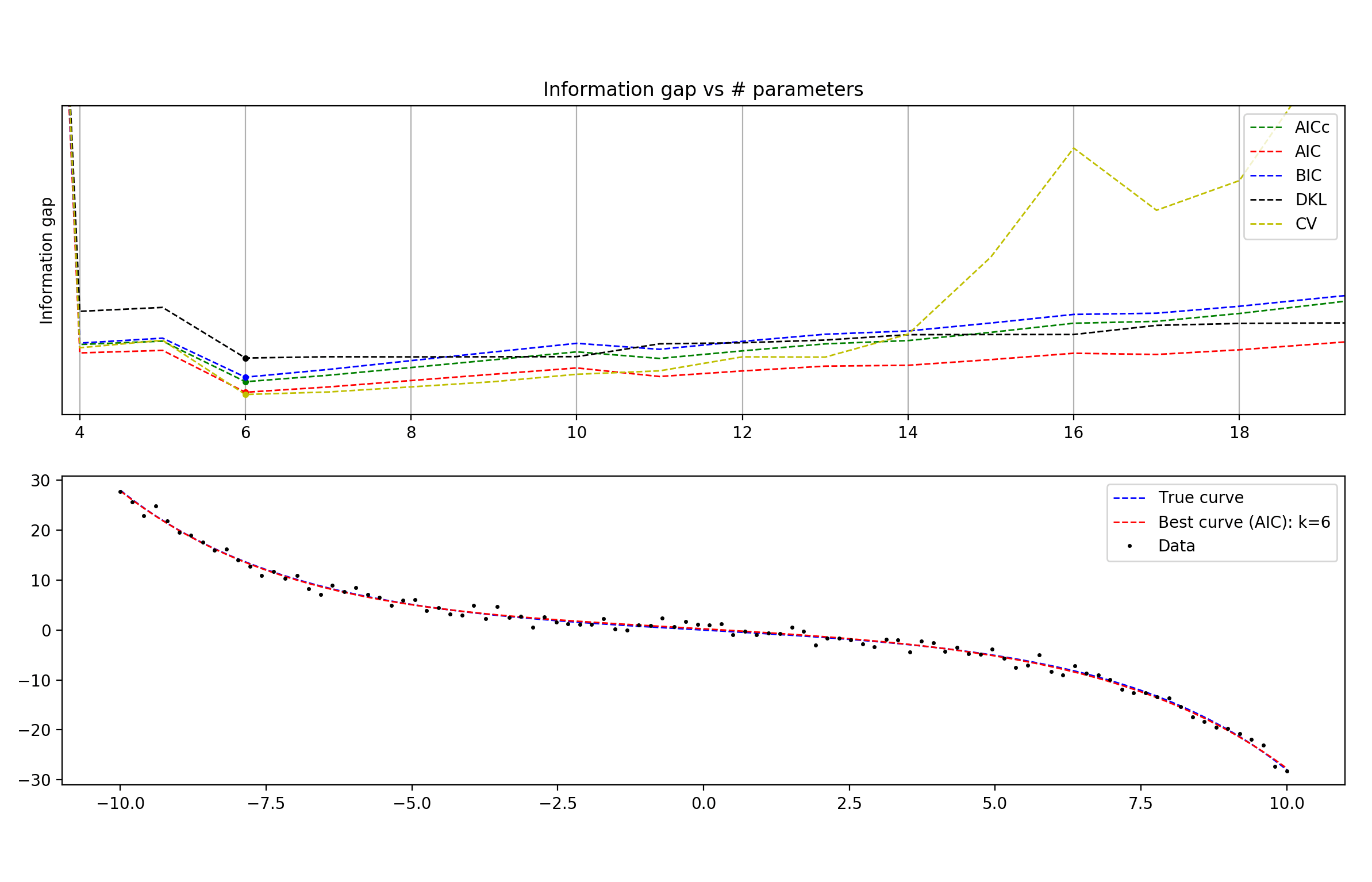For smaller data sets, you can see that AICc tracks DKL much more closely than any other technique (which is of course the entire purpose of AICc).

N = 25N = 25, zoomed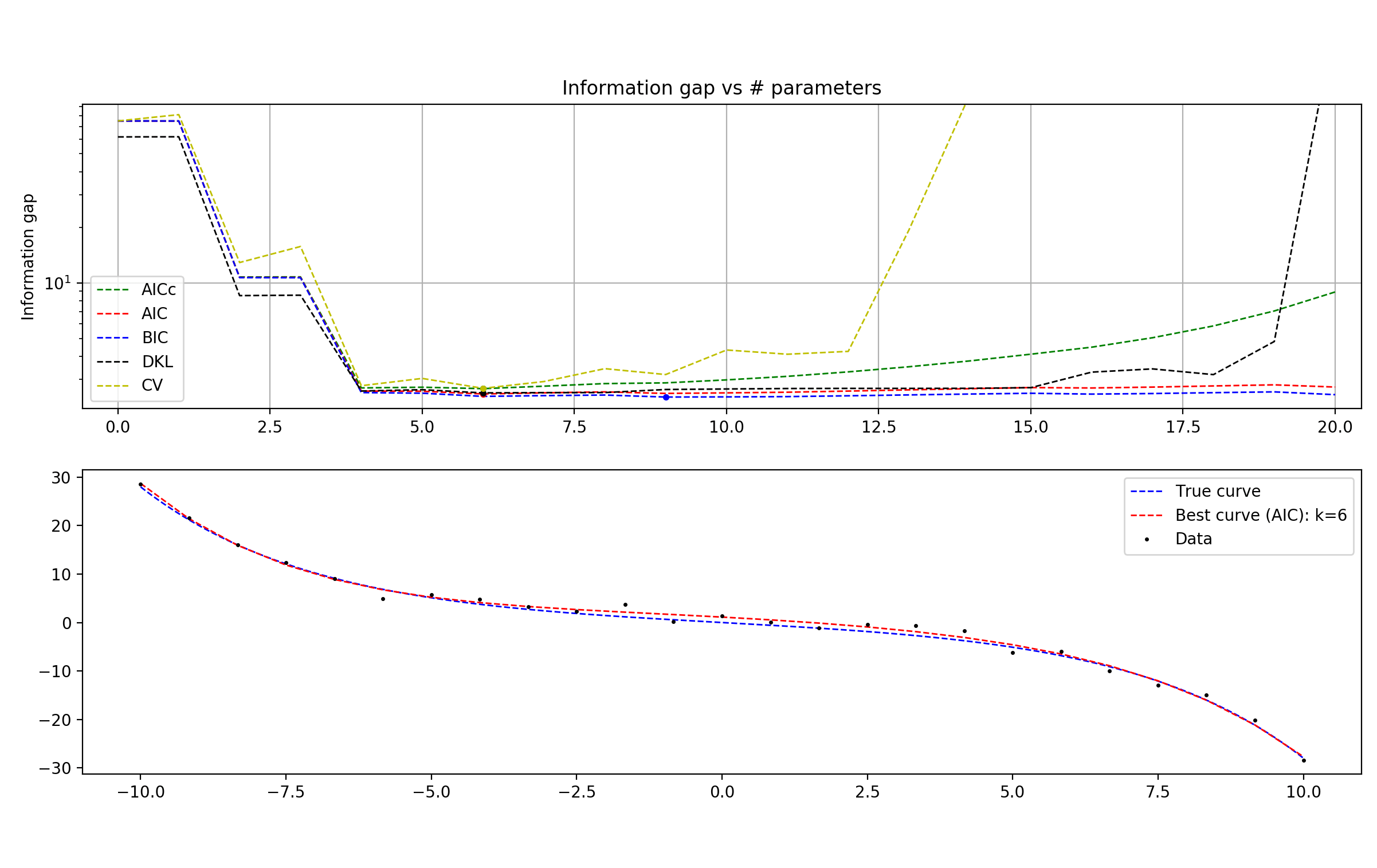N = 25, super zoom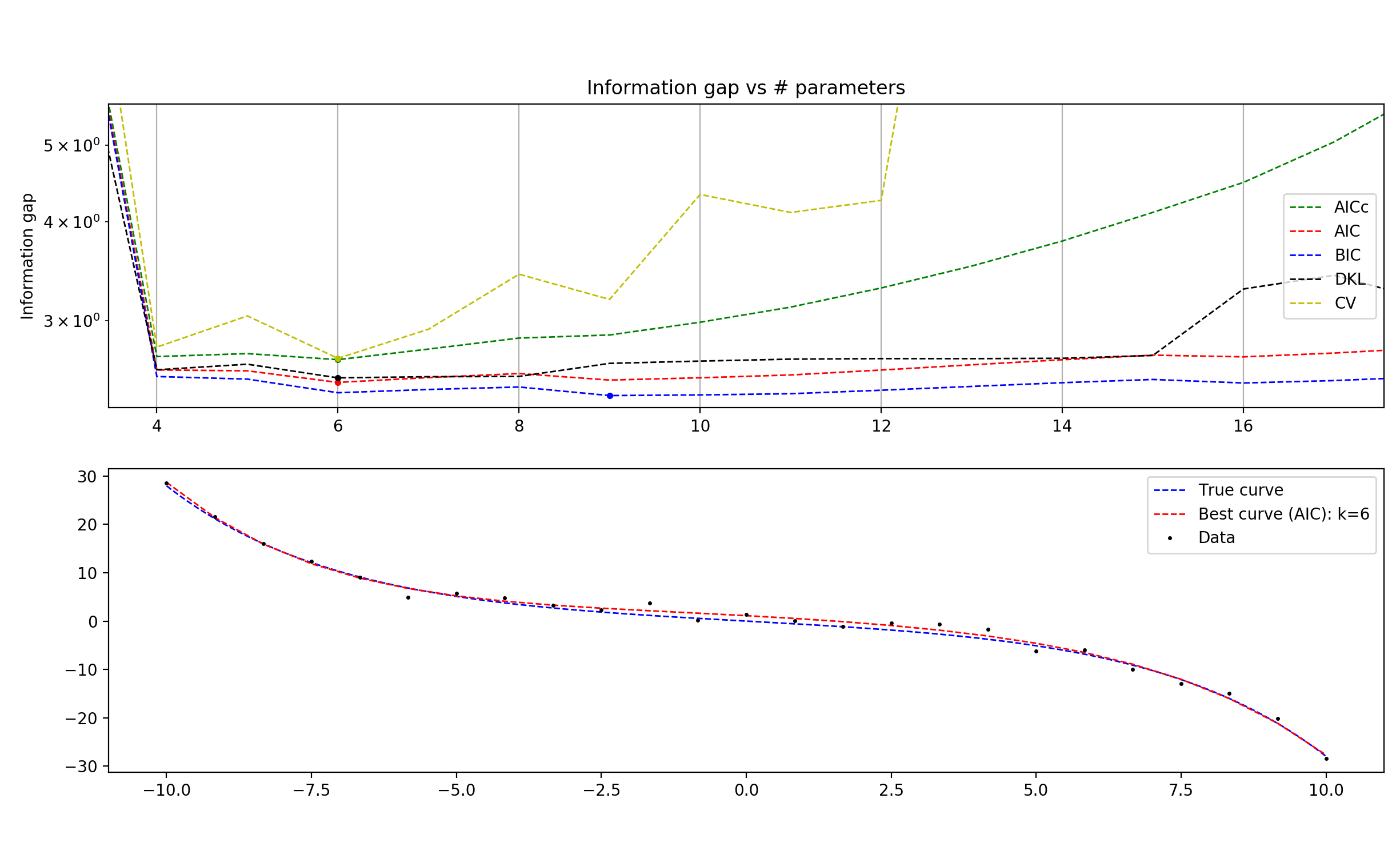Interestingly, you start to see BIC really suffering relative to the other methods, beginning to overfit the data. This is counterintuitive; BIC is supposed to be the method that penalizes complexity excessively and underfits the data. Relevant is that in this program, I use the form of BIC that doesn’t approximate for large N.

BICsmall N = k log(N/2π) – 2L
BICordinary = k log(N) – 2L

When I use the approximation instead, the problem disappears. Of course, this is not too great a solution; why should the large N approximation be necessary for fixing BIC specifically when N is small?

(Edit: after many more runs, it’s looking more like it may have just been a random accident that BIC overfit in the first few runs)

Just for the heck of it, I also decided to torture my polynomials a little bit by making them try to fit the function 1/x. I got dismal results no matter how I tried to tweak the hyper-parameters, which is, well, pretty much what I expected (1/x has no Taylor expansion around 0, for one thing).

More surprisingly, I tried fitting a simple Gaussian curve and again got fairly bad results. The methods disagreed with one another a lot of the time (although weirdly, AICc and BIC seemed to generally be in agreement), and gave curves that were overfitting the data a bit. The part that seems hardest for a polynomial to nail down is the flattened ends of the Gaussian curve.

True curve = 40 exp(-x2/2), N = 100 data pointsAnd zoomed in…If the jaggedness of the cross validation score is not purely an artifact of random fluctuations in the data, I don’t really get it. Why should, for example, a 27-parameter model roughly equal a 25-parameter model in predictive power, but a 26-parameter model be significantly worse than both?